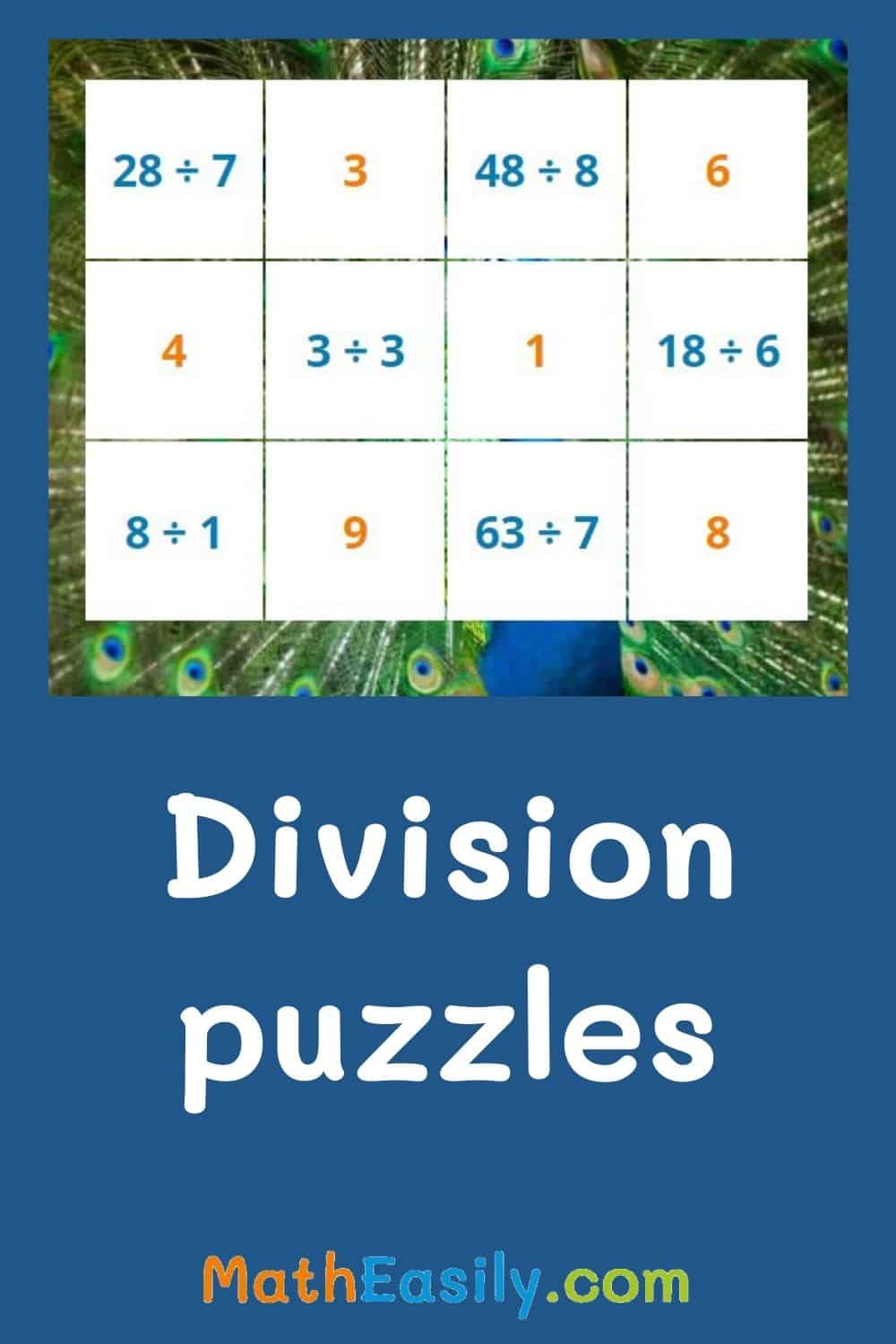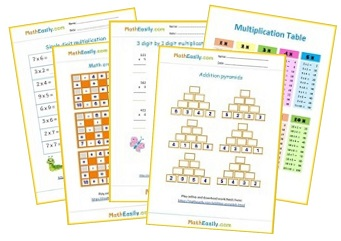﻿ 100+ Free Division Games ONLINE Practice

Page No. 1023

Online Math Games > Division Games

# 100+ Math Division Games Online FREE

Free online division games for kids. Multiplication and division games for the classroom and homeschooling. Play free division math games online, download worksheets.

All these division interactive games and puzzles are accompanied by worksheets on which kids can practice a certain skill.

## Math Division Games Online FREE

### Division puzzles

Learn to divide easily with this online division game. The division puzzle game takes advantage of children's curiosity. As students complete the division tasks, they uncover pictures of animals.
Play now

### Division with pictures

Introduce young learners to the concept of division with this interactive division game. Learn how to perform simple division using pictures for visual representation.
Play now

### Division flash cards

Math division flash cards 1-12 help kids to master basic division. Division card game belongs to our most popular free online division games for kids. It can be played anywhere and anytime.
Play now

### Multiplication and division crossword puzzle

Printable division games: solve mixed multiplication and division crossword puzzle online. Print out the division games in PDF.
Play now

### Missing factor game: BINGO

Click the correct number which can substitute the question mark in the division problem. The object of this game is to get five stars in a row, column or diagonals.
Play now

## Free division games for the classroom

These printable division games complement well with online division games. You can generate as many worksheets as you want, so that every kid in the classroom has own free division game.

Everytime 12 new different division problems are generated and create a new image. More than 50 pictures available.

Click the button and generate the new division game in PDF: Generate

## Multiplication and division games

### Multiplication dice game

Roll a pair of dice, multiply these 2 numbers, check your answer. Play funny multiplication games online.

Play now

### Multiplication flash cards

Online multiplication flash cards (up to 12) help kids to learn multiplication. More than 160 tasks available. Free worksheets.

Play now

### Single digit multiplication

Train single digit multiplication games. More than 81 exercises available. Download worksheets.

Play now

Play now

### 3 digit by 1 digit multiplication

Play now

All multiplication games →

## Printable division games for kids

Download printable division games and worksheets in PDF below. Most of these worksheets have two pages, the first one is the worksheet and the other page contains also the answer key. Browse all our free division worksheets here.## Division Online Games

Online division games for kids improve division and multiplication skills.

Kids will immediately see the correct answer, they can learn from their mistakes. Our free division games spike student engagement, teachers can use them as provoking math activities to start lessons.

Children can play these division games online or you can print them out.

## How to use division math games?

You can explain the concept of division to young students in a simple and interactive way through division games:

1. Introduce the concept: Begin by explaining that division is a way of sharing or grouping things equally. For example, if you have 12 candies and want to divide them among 3 friends, you can show how each friend would get an equal number of candies (4 in this case).
2. Division symbol: Games show the division symbol (÷) and explain that it represents the process of dividing.
3. Define the terms: Introduce the key terms used in division. Explain that the number being divided is called the DIVIDEND, the number by which it is divided is the DIVISOR, and the result of the division is the QUOTIENT.
4. Use visual aids as division puzzles which you can print too. Visual aids can greatly enhance understanding.
5. Practice with simple division problems. You can use division flash cards.
6. Relate division to multiplication: Highlight the connection between division and multiplication. Explain that division is the opposite of multiplication. For example, if 3 friends each received 4 candies, you can show that 3 × 4 = 12 and 12 ÷ 3 = 4.
7. Use real-life scenarios: Help students see the relevance of division in everyday life. Discuss situations like dividing pizza slices among family members or sharing toys among siblings, emphasizing how division helps ensure fairness.
8. Practice and repetition: Provide various division problems for students to solve and encourage them to practice division in different contexts. Gradually increase the complexity of the problems as their understanding improves. Move from basic division problems to division with remainder or to long division.
9. Reinforce learning through printable games or division worksheets: Engage students in division-based games, puzzles, or group activities that make the learning process enjoyable and interactive.Free Math Worksheets